# Greatest Integer Function & Fractional Part Function

## Greatest Integer Function Definition

Greatest Integer Function is defined as the real valued function $f : R \rightarrow R$ , y = [x] for each $x \in R$
For each value of x, f(x) assumes the value of the greatest integer, less than or equal to x.

It is also called the Floor function and step function
Symbol of Greatest Integer Function is []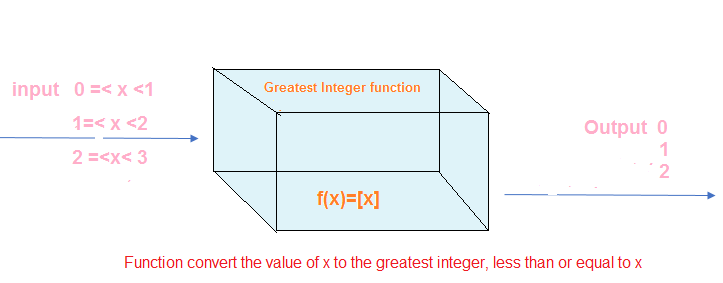Example
[2.56] =2
[.5]=0
[-6.45]=-7
[-.65]=-1

This can be generalized as
$[x] = –1 , –1 \leq x < 0$
$[x] = 0 , 0 \leq x < 1$
$[x] = 1 , 1 \leq x < 2$
$[x] = 2 , 2 \leq x < 3$

Question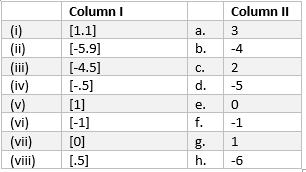## Domain and Range of the Greatest Integer Function

For $f : R \rightarrow R , y = f(x) = [x]$ for each $x \in R$

Domain = R
Range = Z (integer set) as it only attains integer values

## Graph of the Greatest Integer Function

The graph of the Greatest Integer Function is shown below. It coincide with the graph of identity function y=x for $x \geq 0$ and for x< 0, it is a graph of linear function y=-x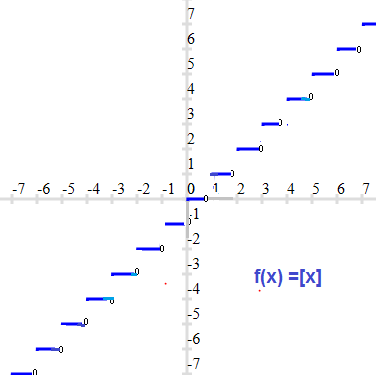## Fractional Part Function(Fractional part of x)

We have seen that
[1.56] =1
[.25]=0
[-5.45]=-6
[-.15]=-1
[-5]=-5
=1
This can be written as
$[x] \leq x$

Fractional part of x is defined as the difference between [x] and x. It is denoted as {x}
So,
{x}=x -[x]
Symbol of Fractional part of x is {} Example
x=4.65 ,then [x]=4 ,{x}=.65
x= -.65, then [x]=-1 ,{x}= .35 ( This is very important thing to remember for negative number)
x =-1.45 ,then [x]=-2 ,{x}= .55 ( This is very important thing to remember for negative number)
x=3 ,then [x]=3 ,{x}=0

We know that
$x-1 < [x] \leq x$
$-x \leq -[x] < 1-x$
$0 \leq x -[x] < 1$
$0 \leq \left \{ x \right \} < 1$

So Fractional part {x} is always non-negative and lies between (0,1).

So we can define Fractional part function as
y=f(x) = {x} =x -[x]

This is good for $x \in R$

Range of the fractional part function is [0,1)
Graph of the fractional part function is given below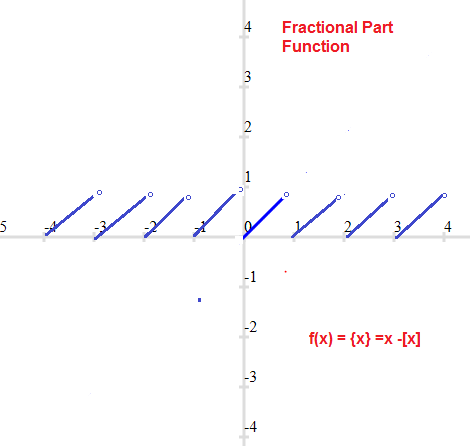Question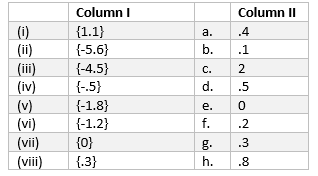## Greatest Integer Function Properties and Fractional part of x Properties

1. If x is an integer

[x]=x
{x} =0

2. For $x \in R$ [[x]]= [x]
[{x}]=0
{[x]}=0

3. for $k \in Z$
a. $[x] \geq k \Rightarrow x \geq n , x \in [n,\infty)$
b. $[x] > k \Rightarrow x \geq n +1 , x \in [n+1,\infty)$
c. $[x] \leq k \Rightarrow x \leq n+1 , x \in (-\infty,n+1)$
d. $[x] < k \Rightarrow x < n , x \in (-\infty,n)$
e. [x+ k]=[x] +k

4.
a. $y=[x]+ [-x]=\begin{cases} 0 & \text{ if } x \in Z \\ -1 & \text{ if } x \notin Z \end{cases}$
b. $y=[x]- [-x]=\begin{cases} 2[x]+1 & \text{ if } x \notin Z \\ 2[x]] & \text{ if } x \in Z \end{cases}$
c. $y=\left\{ x \right\}- \left\{-x\right\}=\begin{cases} 0 & \text{ if } x \in Z \\ 1 & \text{ if } x \notin Z \end{cases}$
3. For real number x and y
a. [x +y] = [x] + [y+x - [x] ]
b. $[x+y]=\begin{cases} [x] + [y] & \text{ if } \left\{x \right\} + \left\{y \right\} < 1 \\ [x]+[y]+1 & \text{ if } \left\{x \right\} + \left\{y \right\} \geq 1 \end{cases}$

## Solved examples of Greatest Integer Function and Fractional part of x

1. Find the domain of the below function?
a. $y =\sqrt {[x] -1|}$
b. $y = \frac {1}{\sqrt { 2- [x]}}$
Solution

a. $y =\sqrt {[x] -1|}$
Clearly This is defined for $[x] -1 \geq 0$
or
$[x] \geq 1$
$x \in [1,\infty)$
So Domain is $[1,\infty)$
b. $y = \frac {1}{\sqrt { 2- [x]}}$
Clearly This is defined for $2- [x] > 0$
or
2 > [x]
or [x] < 2
$x \in (-\infty,2)$
So Domain is $(-\infty,2)$

2. Find the domain and range of function
$y= \frac {1}{\sqrt {x- [x]}}$
Solution
We have ,
$y= \frac {1}{\sqrt {x- [x]}}$
Domain of y
We know that
$0 \leq x -[x] < 1 , x \in R$
for $x \in Z ,x -[x]=0$
So Domain is R - Z
Range of y
$0 < x- [x] < 1 , x \in R -Z$
$0 < \sqrt {x -[x]} < 1$
$1 < \frac {1}{\sqrt {x- [x]}} < \infty$
So Range is = $(1,\infty)$

3. Let $f : R \rightarrow R$ , $f(x) = x^2 + 2[x] -1$ for each $x \in R$
Find the values of f(x) at x= 1.2 ,-.5 ,-2.1
Solution
$f(x) = x^2 + 2[x] -1$
$f(1.2) = (1.2)^2 + 2[1.2] -1 =1.44 +2-1=2.44$
$f(-.5) = (-.5)^2 + 2[-.5] -1 =.25 -2-1=-2.75$
$f(-2.1) = (-2.1)^2 + 2[-2.1] -1 =4.41 -6-1=-2.59$

### Quiz Time for Greatest Integer and Fractional Part Function

Question 1.
Find the domain of the function $f(x)= \frac {1}{\sqrt {[x]-x}}$
A. R - Z
B. R
C. {}
D. Z
Question 2.
Find the range of values of x for which $[x]^2 -4 =0$ $A.$[-2,-1) \cup [2,3)$B.$[-2,-1] \cup [2,3]$C.$[-2,-3) \cup [2,3)$D.$[-2,-3] \cup [2,3)$Question 3. if$f(x) =[x]^2 +[x] +2$,then which of them is incorrect A. f(1)=4 B. f(.75)=2 C. f(-.50)=2 D. f(0) =3 Question 4. Value of the expression [{x}] + {[y]} is A. 1 B. 0 C. 2 D. Cannot be determined Question 5. The value {-.75} where {.} is the fractional part is A. -.75 B. .25 C. 0 D. None of these Question 6. The domain of the function f given by$f (x) = \frac {1}{\sqrt {x^2 -[x]^2}}\$ is
A. Domain = R
B. Domain = R+
C. Domain = R+ -Z
D. Domain = Z